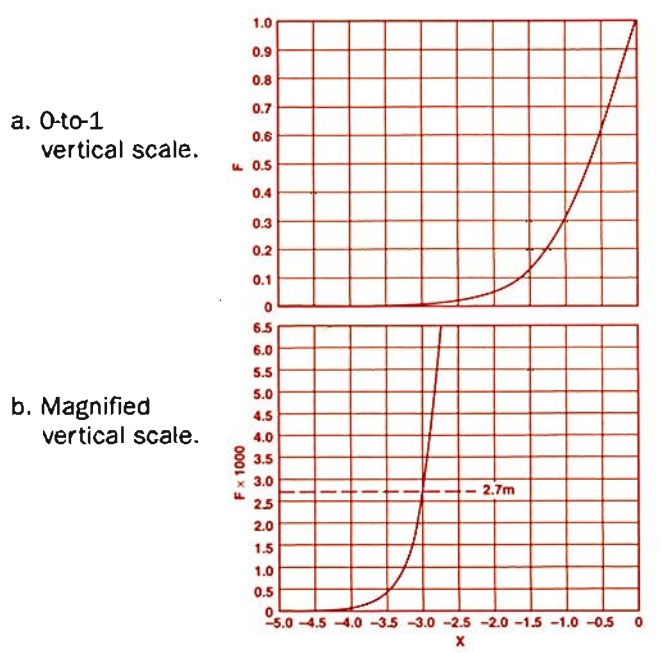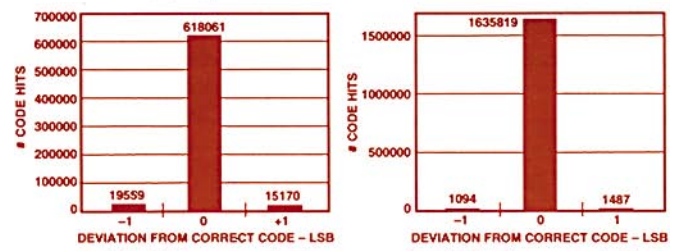# Using Histogram Techniques to Measure A/D Converter Noise

This article describes a technique for using histograms to determine the rms noise of an A/D converter, referred to the input. It can complement the popular qualitative approach of evaluating A/D converter performance by applying a dc analog voltage and observing "code flicker" at the A/D's digital output.

Collect Data With A Histogram: A dc histogram reports the number of times each code appears at the output of the A/D converter. Here is a procedure to produce a histogram:

• Connect a "clean" dc source to the analog input of the A/D converter (and a "clean" reference, if an external one is used).
• Record the results of a large number of conversions, depending on the expected noise. One-to-two million conversions are usually more than adequate for a low-noise A/D.
• Sort the conversions into code "hits." The core of the histogram is essentially a bank of counters (or bins); there is one bin corresponding to each possible digital output of the A/D converter. After each conversion, the digital code at the A/D output is determined (by hardware or software) and the corresponding counter is incremented. If an ideal (noiseless) A/D were connected to a dc voltage, the histogram of n conversions would indicate n code 'hits' in the bin corresponding to the digital value of the dc input. All other bins would equal zero.

While the ideal A/D only produces code hits in a single bin, an actual A/D will produce additional codes outside the main bin for any dc input value, due to the presence of noise. The number of codes that fall outside the main bin is the key to measuring the converter's rms noise. Avoidance of an additional effect at certain codes due to differential nonlinearity will be discussed later.Figure 1. Quantization of a probability distribution.

Understand Noise Sources: The three dominant sources of A/D noise are quantization, ac (phase noise, or jitter in sampling of ac signals), and wideband (white noise, digital feedthrough, etc.). The last one is the most amenable to dc histogram testing. Quantization noise is a large contributor to the signal's error power, but (in the absence of drift during the measurement) it does not cause multiple code "hits" to show up in a dc histogram. AC noise, resulting from aperture jitter, is also not a factor when the input signal is dc. The remaining source, wideband noise, is the major input-referred noise component measured by the histogram technique.

What are our important assumptions? First, that the model for the A/D is an ideal quantizer with a Gaussian noise source added to the input of the device. The measurement will be somewhat erroneous if the dominant noise source is non-Gaussian; for example, digital feedthrough tends to be code and signal dependent. Second, the architecture of the device affects the measurements; but the technique is valid for the majority of high-speed, pipelined converters available from Analog Devices.

Determine RMS Noise Graphically: How do the histogram and noise relate to one another? Figure 1 shows a Gaussian input to an ideal quantizer and a histogram of the corresponding output. The probability density function (PDF) of the input shows that the majority of the output codes will occur in a single bin, but there must be additional codes corresponding to the tails of the distribution. The fraction that occurs outside the main code depends on the spread, or standard deviation, σ, of the noise distribution.

Figure 2 is a plot of a PDF for A/D input noise in which σ corresponds to the least significant bit (LSB) of the converter under test. The dc input to the A/D is adjusted to center the PDF on the midpoint of code k. After quantization, noise is measured by the fraction of codes occurring outside the main bin, equal to the ratio of the area of the shaded tails to the total area under the curve. With the analog input on a code center, the boundaries of the shaded area occur at ±0.5 LSB away from the code center.Figure 2. A Gaussian distribution scaled in LSBs, about the mean.

For a symmetrical Gaussian PDF, the equation1,

expresses the probability of getting a code outside the main bin for that PDF. The terms inside the integral are simply the expression for a Gaussian distribution with unit area and standard deviation, σ. Integrate from -infinity to -0.5 LSB to find the area under one tail, and from +0.5 LSB to +infinity for the other. By symmetry, one can simply multiply the area under one tail by 2.

To ultimately determine the A/D's noise, equation (1) must be solved for σ, with P determined from the histogram as the fractional portion of code "hits" outside the main bin. Unfortunately, the integral has no closed form solution in terms of σ. One option is to iterate σ and numerically evaluate equation (1) until the solution equals the fraction determined from the histogram. Alternatively, we can fix σ, iterate the upper limit of integration, plot the result as a graph, and use it to find the upper limit that corresponds to any value of P. Figure 3a is a plot of:

with σ equal to unity. For each point, x, it has been integrated and the fractional value, F, determined. Since we already know the value of F (= P) from the histogram, we can simply find the x-axis point, x0, corresponding to P, from Figure 3a. This value is equivalent to having evaluated equation (1) from -infinity to -0.5 LSB, had we known the correct value for σ. To calculate the rms noise of the converter, simply solve the equation

The procedure's simplicity can be demonstrated with an example. Suppose that the fraction of codes outside the main bin equals 0.0027. Using Figure 3b, a magnified version of 3a, find 0.0027 on the y-axis [these values can also be found from a standard normal curve, or z-Table for the area under the unit normal distribution]. The corresponding intercept on the x-axis, x0, is -3. Solve equation (3) for σ, the rms noise (= 0.167 LSB).

Figures 3a and 3b relate rms noise and code histogram values. Do a histogram, find the fraction of codes that occur outside the main bin, look up that number on the y-axis of 3a or 3b, and read off the x-axis value. Dividing 0.5 LSBs by this number gives the rms noise in LSBs.Figure 3. Plots of F vs. X for equation (2)

On The Bench: There are some practical rules to follow when setting up the test fixture. First, use a dc source with noise below the level being measured. Then, using several conversions, set the analog input squarely on the center of the code (i.e., mid-way between adjacent transitions). This should make the side bins approximately equal in size, important for the symmetry assumption in using the above analysis. This also maximizes the number of code hits occurring outside the main bin(s), resulting in a statistically more-significant measurement. For example, if the input is centered on the transition, the histogram produces two 1-LSB-wide main bins with approximately equal numbers of code "hits", including some of the hits that would have appeared in the bins adjacent to a 1-LSB-wide centered main bin.

Determining the noise of the converter is useful for making a comparison, either to a specified requirement or to another A/D converter of the same, or different type. Figure 4a shows a typical histogram generated from the AD872A 12-bit, 10-MSPS A/D converter. All the codes appear in either the main bin or the two adjacent bins, roughly symmetrically disposed. From the histogram, the ratio of the 34,729 code hits outside the main bin to the 652,790 total number of codes counted is 0.053. From Figure 3a, the x-value corresponding to 0.053 on the y-axis is approximately 1.9 σ. From equation (3), 1.9 σ = 0.5 LSBs, and the rms noise (σ) equals 0.26 LSBs rms.a. AD672A, sampled at 10 MSPS. b. AD871, sampled at 5MSPS.

For comparison, Figure 4b shows a histogram of the AD871, a 12-bit, 5-MSPS high-speed A/D converter. Using the same procedure, the hit ratio is 2,581/1,638,400, about 0.0016, and the input-referred noise of the AD871 is thus 0.5/3.17, or 0.16 LSBs rms. With low-noise A/Ds, like the AD871, the test should be run long enough to acquire enough code hits outside the main bin in order to obtain a credible noise measurement.

DNL May Skew The Measurement: While the histogram measurement technique assumes an ideal quantizer, differential-nonlinearity (DNL) errors will influence the measurement. DNL, a measure of A/D converter code width, expresses the difference between an actual code width and the ideal width of 1 LSB; it is positive for a wide code, negative for a narrow code. It tends to be worst at major transitions, e.g., from 011...11 to 100...00. If the code is wide, the noise measurement will be optimistic because the main bin will contain more hits. If a code is narrow, the noise measurement will tend to be on the high side (however, since missed codes are the ultimate case of excessively narrow codes, the measurement is conservative). The easiest way to circumvent this problem is to compute σ at several points along the transfer function avoiding major transitions, wide codes, and narrow codes—and then average the results.

### Ссылки

1Drake, Alvin W., Fundamentals of Applied Probability Theory. McGraw-Hill Book Company, 1967